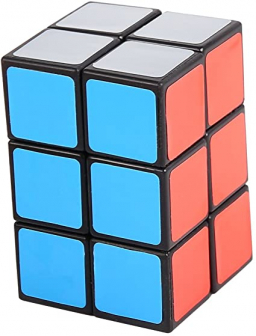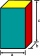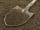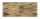# A dumpster

A dumpster is shaped like a rectangular prism. It has a width of 8 feet, a height of 5 feet, and a volume of 880 cubic feet.

What is the length of the dumpster?

l =  22 ft

### Step-by-step explanation:Did you find an error or inaccuracy? Feel free to write us. Thank you!Tips to related online calculators
Tip: Our volume units converter will help you with the conversion of volume units.

## Related math problems and questions:

• Water tankWhat is the height of the cuboid-shaped tank with the bottom dimensions of 80 cm and 50 cm if the 480 liters of water reach 10 cm below the top?
• Reservoir 3How many cubic feet of water is stored in the reservoir that has a length of 200 feet, a width of 100 feet, an overflow depth of 32 feet, and a current water level of 24 feet?
• FloorThe rectangular floor of the living room has a length of 5.4 meters and a circumference of 17.2 meters. What is its width?
• Four prismsQuestion No. 1: The prism has the dimensions a = 2.5 cm, b = 100 mm, c = 12 cm. What is its volume? a) 3000 cm2 b) 300 cm2 c) 3000 cm3 d) 300 cm3 Question No.2: The prism base is a rhombus with a side length of 30 cm and a height of 27 cm. The height of t
• Rectangular prismIf I have a rectangular prism with a length of 1,000 cm, a width of 30 cm, and a height of 50 cm, what is the volume?
• Third dimensionCalculate the third dimension of the cuboid: a) V = 224 m3, a = 7 m, b = 4 m b) V = 216 dm3, a = 9 dm, c = 4 dm
• Edges of the cuboidFind the length of the edges of the cuboid, which has the following dimensions: width is 0.4 m; the height is 5.8 dm and the block can hold 81.2 liters of fluid.
• Height of the cylinderThe cylinder volume is 150 dm cubic, the base diameter is 100 cm. What is the height of the cylinder?
• Cuboid heightWhat is the height of the cuboid if the edges of its base are 15 cm and 4 cm long and its volume is 420 cm cubic?
• ClayHow many cubic centimeters of clay is in a pit of dimensions 4 m x 3 m x 3 m?
• Dana helpedDana helped her dad build a sandbox for her younger sister. The sandbox is shaped like a rectangular prism that is 4 1/2 feet long and 4 feet wide. Dana used bags of sand to fill the sandbox 1/2 of a foot deep. Each bag contained 1/2 of a cubic foot of sa
• PrismThe base of the prism is a rhombus with a side 30 cm and a height 27 cm long. The height of the prism is 180% longer than the side length of the rhombus. Calculate the volume of the prism.The quadrangular prism has a volume of 648 cm3. Trapezoid which is its base has the dimensions bases: a = 10 cm, c = 5 and height v = 6 cm. What is the height of the prism?The block-shaped flowerpot has external dimensions: length 1.25 m, width 10 cm, and height 11 cm. The thickness of the boards from which it is made is 0.8 cm. How many liters of soil is needed to fill it 1 cm below the top edge? What surface do we have toFind the surface of the cuboid when its volume is 52.8 cubic centimeters, and the length of its two edges is 2 centimeters and 6 centimeters.The base of the perpendicular prism is a rectangular triangle whose legs length are at a 3: 4 ratio. The height of the prism is 2cm smaller than the larger base leg. Determine the volume of the prism if its surface is 468 cm2.The volume of tetrahedral prism is 2.43 m3. Base of prism is a parallelogram in which a side 2,5dm and height ha = 18cm. Calculate the height of the prism.